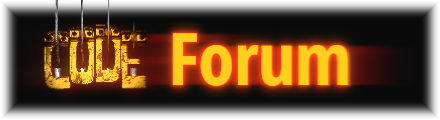# Staff notation question: notating varying subdivisions

Your post makes perfect sense to me for the 12/8 and 16/8 times, but I can’t see how 16/8 becomes 4/4.

And this got me wondering: Is 12/8 == (6x2)/(4x2) == 6/4?

And is 16/8 == (8x2)/(4x2) == 8/4?

Sorry to ask such fundamental questions but I can’t help myself.

Sixteen 8th notes would normally make two bars of 4/4 which is why I presented that as an option too. Even though 16/8 is a silly time signature, it indicates that there are four more 8th notes than the other bars.

12/8 is not the same as 6/4 in the same way that fractions would be in math. I’ll try to come back and explain when I have more time.

In music, the magic equation to transform simple signatures into compound is to multiply and/or divide time signatures by 3/2. Keeping ‘why’ aside for a second, the time signature twins you’re looking for, are:

2/4, simple time signature - as compound, it becomes 2/4*3/2 = 6/8

Following the same thought process:

3/4 = 9/8

4/4 = 12/8

6/4 as compound would be 18/8

Compound time signatures are broken down into three-part rhythms.

The reason 16/8 equals 4/4 in the given situation is because both are SIMPLE time signatures, that means that they both can be broken down in a two-part rhythm. The first cue you should note is how the 8th notes are grouped together. 4/4 and 16/8 are basically the same visually speaking, the only difference would be how you count them: instead of 1-and-2-and-and-4-and, you would count 1, 2, 3, 4, 5… 16! in the 16/8 situation. That means 16/8 is overkill and unecessary, but just a reminder of what is possible. 16/8 is the literal description of how many 8th notes are in two measures of 4/4.

1 Like

So I see that 16/8 == 8/8 + 8/8 that can be viewed as 4/4 except with TWICE the bars; OK.

But if somebody can explain the 3/2 business I would be most thankful!

1 Like

I’ll try my best:

First, start a metronome on 120 bpm.

To explain the multiplier you have to keep in mind what a time signature means. What is 4/4?

The lower number represents the figure of your pulse. That means you should hear the constant sound of a metronome as a quarter note. The upper number represents the amount of quarter notes you can fit in a measure (in this case, 1, 2, 3, 4).

When you multiply the lower number by 2, the constant pulse of a metronome should now be heard as eighth notes (1 and 2 and). The reason why the counting stopped at the fourth pulse is because we only multiplied the lower number by two, that means we only have 4/8 (only 4 eighth notes are allowed). Multiply the numerator by 2, you get 8/8 (1 and 2 and 3 and 4 and). Even though using eighth notes as a denominator in a simple time signature is kinda rare, it can happen. Stop the metronome. Example of 8/8 in ‘real life’:

This song mixes 7/8, 8/8 and many others. Always keeping the eighth note pulse throughout the whole song, if I’m not mistaken.

Start the metronome again.

The number 3 comes in when you want a different feel to the pulse (that’s why we call it “compound”). That means, instead of a two-part rhythms like before (4 turning into 8). You want a valselike feel to the pulse. Valses have a three-part rhythm. It gives a ‘dragging’ type of feel (4 turns into 12). You know when you see a triplet in 4/4? That’s the default eighth notes for 12/8!! (Ex 2b) The reason we notate them with a number 3 on top (in simple signatures) is to give the musician the understanding that you have a compound feel for just a brief moment.